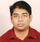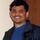Cloud Insights

## In Cloud Secure, how to specify the forest name while adding the LDAP Data Source Collector1,921 Views

In Cloud Secure can I provide the forest name in comma format (dc=x,dc=y,dc=z) or do I have to provide in x.y.z format?

How do I add a single user from the forest in CloudSecure while doing testing?

Regards,

Abhi

1 ACCEPTED SOLUTION1,897 Views

Yes you can.

Forest name allows both formats.

• x.y.z => direct domain name as you have it on your SVM. [Example: hq.netapp.com]
• DC=x,DC=y,DC=z => Relative distinguished names [Example: DC=hq,DC=netapp,DC=com]

You can even specify like below

• OU=engineering,DC=hq,DC=netapp,DC=com [filter by specific OU engineering]
• CN=sandeep,OU=engineering,DC=hq, DC=netapp, DC=com [specific user sandeep OU engineering]
• CN=Acrobat Users,CN=Users,DC=hq,DC=netapp,DC=com,O=NetApp,L=Waltham,S=MA,C=US [all Acrobat users in the filters applied]1,898 Views

Yes you can.

Forest name allows both formats.

• x.y.z => direct domain name as you have it on your SVM. [Example: hq.netapp.com]
• DC=x,DC=y,DC=z => Relative distinguished names [Example: DC=hq,DC=netapp,DC=com]

You can even specify like below

• OU=engineering,DC=hq,DC=netapp,DC=com [filter by specific OU engineering]
• CN=sandeep,OU=engineering,DC=hq, DC=netapp, DC=com [specific user sandeep OU engineering]
• CN=Acrobat Users,CN=Users,DC=hq,DC=netapp,DC=com,O=NetApp,L=Waltham,S=MA,C=US [all Acrobat users in the filters applied]